Next: 1.3 Thermoelectric Materials Up: 1. Introduction Previous: 1.1 Fundamentals of Thermoelectrics   Contents

# 1.2 Device Efficiency

The efficiency of thermoelectric devices in converting heat into the electricity is defined as the ratio of energy provided to the external load'' to heat energy absorbed at the hot junction''. The maximum efficiency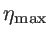of the power generator is achieved when the external load is matched with the device resistance :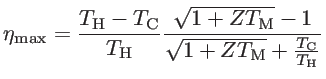(1.8)

Here,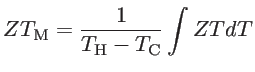(1.9)

is the average of the thermoelectric device figure of merit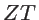defined as: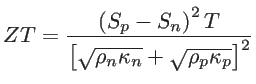(1.10)

where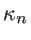and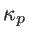are the thermal conductivities of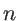-type and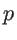-type materials, respectively.

The efficiency of a thermoelectric power generator, as any other heat engine, is less than the Carnot engine efficiency: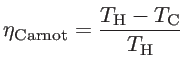(1.11)

Indeed, the Carnot engine has the most efficient cycle for converting a given amount of thermal energy into work. In addition to the temperature difference,is also related to the average of, which is related to the material properties of the-type and-type materials used in the thermoelectric element. Figure 1.5 shows the maximum efficiency of the thermoelectric generator as a function of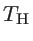for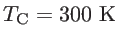and different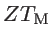. The efficiency increases withand. For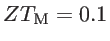, the maximum efficiency is about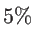. The efficiency for highincreases to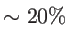and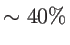for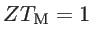and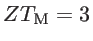, respectively. The efficiency of Carnot cycle is plotted as well for comparison. We also note that the efficiency of some other common energy convertors is as follows: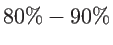for hydro-electric technologies,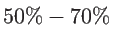for fuel cells,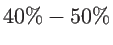for wind turbines, and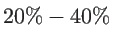for tidal turbines . As a result, an average figure of merit higher than 3 is required, in order to compete with the rest of the commercial generators that are already in large-scale use in the market .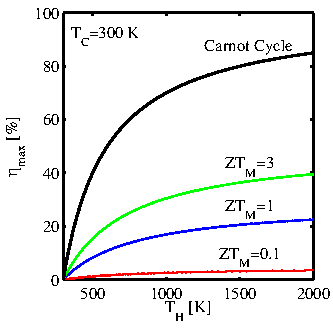The thermoelectric device figure of merit and the efficiency are related to the material properties of the-type and-type semiconductors as well as the electrical and thermal contact resistances. For simplicity, the thermoelectric material figure of merit: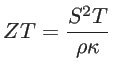(1.12)

can provide an approximation for the thermoelectric figure of merit. The Eq. 1.12 can be alternatively written in terms of the electrical conductance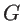and thermal conductance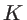as: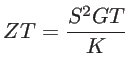(1.13)

However, one should note that to achieve a high efficiency, it is necessary to have both- and-type materials with high averageover a wide range of temperatures .Next: 1.3 Thermoelectric Materials Up: 1. Introduction Previous: 1.1 Fundamentals of Thermoelectrics   Contents
H. Karamitaheri: Thermal and Thermoelectric Properties of Nanostructures